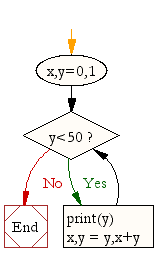## ~ OOP,Classes,Python,Tkinter ...recreating PONG

### Code and Challenge

Copy and paste the code below. Run it! Read the task and see if you can do it before moving on to the next challenge

```"""

Can you draw what is happening between the ball and the bat and explain the collision detection

NOTE OF POSITIONS

ball pos  Top left coordinate x1
ball pos  Top left coordinate y1
ball pos  Bottom right coordinate x2
ball pos  Bottom right coordinate y2

bat pos  Top left coordinate x1
bat pos  Top left coordinate y1
bat pos  Bottom right coordinate x2
bat pos  Bottom right coordinate y2

"""

from tkinter import *
import random
import time

class Ball:
def __init__(self,canvas,bat,color):  #change the __init__function in class Ball to pass in the bat object as a parameter
self.canvas=canvas
self.bat=bat #here we assign the bat parameter to the object variable bat
self.id=canvas.create_oval(30,30,50,50,fill=color)
self.canvas.move(self.id,100,200)
starting_position=[-3,-2,-1,1,2,3]
random.shuffle(starting_position)
self.x = starting_position
self.y = -3
self.canvas_height=self.canvas.winfo_height()
self.canvas_width=self.canvas.winfo_width()

#Create the hit_bat function
def hit_bat(self,pos):
bat_pos=self.canvas.coords(self.bat.id) #retrieve the coordinates of the bat position - note the ball coordinates are being passed
if pos >=bat_pos and pos <=bat_pos: #if the right side of the ball (that is the x right hand coordinate) is greater than the left side of the bat, AND the left side of the ball is less than the right side of the bat ....move etc
if pos>=bat_pos and pos <= bat_pos: #if the bottom of the ball (pos) is between the paddle's top [bat pos) and bottom (pos)
return True
return False

def draw(self):
self.canvas.move(self.id,self.x,self.y)
pos=self.canvas.coords(self.id)
if pos <=0:
self.y=6
if pos >=self.canvas_height:
self.y=-6
#Call the hit_bat function
if self.hit_bat(pos) ==True: #if the hit_bat function returns a value of True, the direction of the ball is changed
self.y=-6 #if the ball hits the bat, then send the ball flying up at a rate of -6 (higher the number the faster the fly!)
if pos <=0:
self.x=6
if pos>=self.canvas_width:
self.x=-6

class Pongbat():
def __init__(self,canvas,color):
self.canvas=canvas
self.id=canvas.create_rectangle(0,0,100,10,fill=color)
self.canvas.move(self.id,200,300)
self.x=0
self.canvas_width=self.canvas.winfo_width()
self.canvas.bind_all('',self.left_turn)
self.canvas.bind_all('',self.right_turn)

def draw(self):
self.canvas.move(self.id,self.x,0)
pos=self.canvas.coords(self.id)
if pos<=0:
self.x=0
if pos>=self.canvas_width:
self.x=0

def left_turn(self,evt):
self.x=-10

def right_turn(self,evt):
self.x=10

def main():
tk=Tk()
tk.title("My 21st Century Pong Game")
tk.resizable(0,0)
tk.wm_attributes("-topmost",1)
canvas=Canvas(tk,bg="white",width=500,height=400,bd=0,highlightthickness=0)
canvas.pack()
tk.update()

bat1=Pongbat(canvas,'red') #this has to be created first, otherwise it will not recognise the call to the bat parameter in the next statement
ball1=Ball(canvas,bat1, 'green') #change this so that it includes the bat parameter (we included it in the ball's __init__method

while 1:
tk.update()
ball1.draw()
bat1.draw()
time.sleep(0.02)
main()

#note - an ERROR may be caused as Python is complaining about breaking out of the while loop when the window is closed - you can ignore that for now (or a fix is provied in the solutions section for those who are interested"""

```

Systems Life Cycle (in a nutshell): Analyse - Design - Create - Test - Evaluate. Designing something or writing out some pseudocode before you actually write code is always a good idea! Get in to the habit of doing so! You can draw your flowchart here and screenshot it.

A sample flow chart (design) for this particular challenge could look like:Each challenge section below provides an online drawing tool where you can dynamically create flowcharts. Screenshot them into your presentation for submission.